#Function Repository Resource:

# SameAsQ

An operator form of SameQ

Contributed by: Richard Hennigan (Wolfram Research)
 ResourceFunction["SameAsQ"][this][that] yields True if the expression that is literally this, and yields False otherwise. ResourceFunction["SameAsQ"][x1,x2,…][y1,y2,…] is equivalent to SameQ[x1,x2,…,y1,y2,…].

## Details and Options

ResourceFunction["SameAsQ"] requires exact correspondence between expressions, except that it still considers Real numbers equal if they differ in their last binary digit.
ResourceFunction["SameAsQ"][2.] gives False.
ResourceFunction["SameAsQ"][] is equivalent to SameQ.
ResourceFunction["SameAsQ"][][] is equivalent to SameQ[].

## Examples

### Basic Examples (3)

Test whether two expressions are identically the same:

 In:=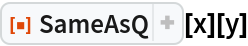Out=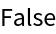In:=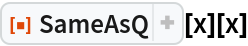Out=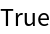Separate a specific element from a list:

 In:=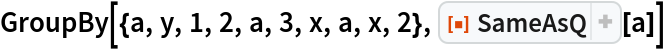Out=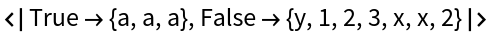Test equivalence of strings:

 In:=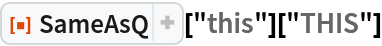Out=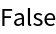### Scope (3)

Numbers in different representations are not the same:

 In:=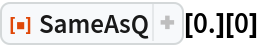Out=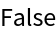== nevertheless treats them as equal:

 In:=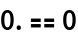Out=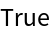Approximate numbers that differ in their last bit are still considered identical:

 In:=Out=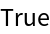Compare unevaluated expressions:

 In:=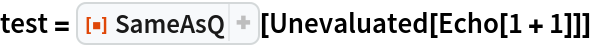Out=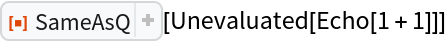In:=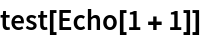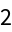Out=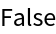In:=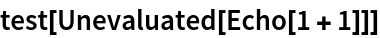Out=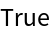Without the Unevaluated, expressions will be compared with 2:

 In:=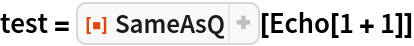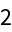Out=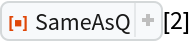In:=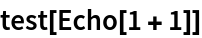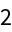Out=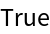In:=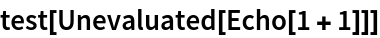Out=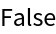### Properties and Relations (4)

SameQ cannot have an operator form, since a single element is the same as itself:

 In:=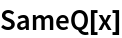Out=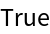SameAsQ can be used as an operator form for SameQ:

 In:=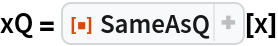Out=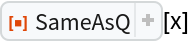In:=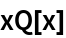Out=In:=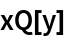Out=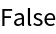Multiple arguments can be given in either position:

 In:=Out=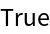In:=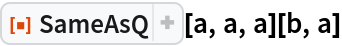Out=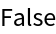This function will always return False:

 In:=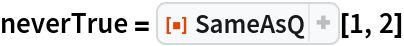Out=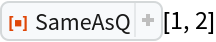In:=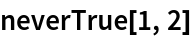Out=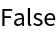In:=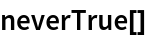Out=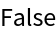This is effectively equivalent to the following:

 In:=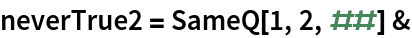Out=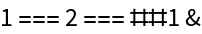In:=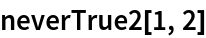Out=In:=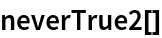Out=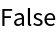Absolutely nothing is the same as absolutely nothing:

 In:=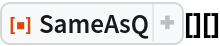Out=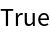This is equivalent:

 In:=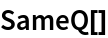Out=### Applications (2)

Create FalseQ, a variant of TrueQ that does what you probably expect:

 In:=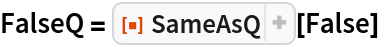Out=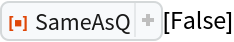In:=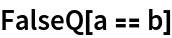Out=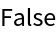In:=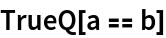Out=In:=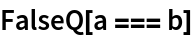Out=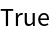In:=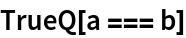Out=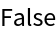In:=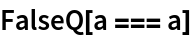Out=In:=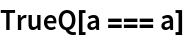Out=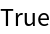Compare:

 In:=Out=## Requirements

Wolfram Language 11.3 (March 2018) or above

## Version History

• 1.0.0 – 20 March 2019